# Book: 20180207Attempt

My answer on February 7, 2018

I will share some progress that I am making in answering my own question. It comes from studying Victor Kac's lecture notes, and in particular, Lecture 15, and thinking concretely about how the simple roots arise from pairs of the diagonal entries of the matrices of the Cartan subalgebra. Perhaps somebody will have further or better thoughts. Basically, I may have found the metaphysical or cognitive bottleneck (in the good sense) that I am looking for, but now I need to link that to meaningful constraints on Lie Algebras and Lie Groups.

Here is the closest that I seem to get to the crux. Imagine a counting process {$1 \rightarrow 2 \rightarrow 3 \rightarrow \dots n-1 \rightarrow n$} which we encode algebraically as {$x_{1}-x_{2}, x_{2}-x_{3}, \dots , x_{n-1}-x_{n}$}. All of the classical Lie algebras share this set of simple roots. We can think of this as a signal propagating forward. The classical Lie algebras are distinguished by one additional simple root for which there are four allowed possibilities:

{$\begin{array}{1|2]3|4} A_{n} & n \rightarrow n+1 & x_{n}-x_{n+1} & = x_{n}-x_{n+1} \\ B_{n} & n \rightarrow 0 & x_{n}-0 & = x_{n} \\ C_{n} & n \rightarrow -n & x_{n}- -x_{n} & = 2x_{n} \\ D_{n} & n \rightarrow -(n-1) & x_{n}- -x_{n-1} & = x_{n-1}+x_{n} \\ \end{array}$}

I interpret {$A_{n}$} as the possibility that the sequence continues propagating forward. Otherwise the sequence is reflected backward. The sequence can take us first to an external mirror {$0$} from which we would proceed to count backwards {$-x_{n},-x_{n-1},\dots,-x_{1}$}. Or the sequence can take us from {$x_{n}$} directly to its reflection {$-x_{n}$}. Or it can turn out that {$x_{n}$} is itself a mirror and so the counting proceeds {$x_{n-1} \rightarrow x_{n} \rightarrow -x_{n-1}$} where we may think of {$x_{n}$} and {$-x_{n}$} as intrinsically indistinguishable conjugates.

The point is that there is a duality between counting forwards and counting backwards. (I imagine it as an outside view, watching the count forwards, like a tube growing, and then an inside view, where we go inside the sequence, counting backwards.) So long as we are counting forwards, the duality is potential. Once we start counting backwards, then the duality is manifest. And there are three ways that it can manifest itself: with an external mirror {$0 \leftrightarrow -0$}, without an explicit mirror, and with an internal mirror {$x_{n} \leftrightarrow -x_{n}$}.

This is a rather straightforward analysis of the options for the diagonal entries of the Cartan subalgebra in Victor Kac's Lecture 15. The adjoint representation is relating pairs of these entries. The one subtle point is that in the case of the orthogonal Lie algebras {$B_{n}$} and {$D_{n}$} the matrices in the algebras have zeros on their anti-diagonal with the consequence that the adjoint representation never pairs {$x_{i}$} with {$-x_{i}$}. But {$B_{n}$} gets around this because it is odd-dimensional and so has a diagonal element {$x_{n+1}=-x_{n+1}=0$} and thus can pair {$x_{n}$} and {$0$} yielding the simple root {$x_{n}-0$}. For this reason {$B_{n}$} is similar to the symplectic algebra {$C_{n}$} which can pair {$x_{n}$} and {$-x_{n}$} directly yielding the simple root {$x_{n}- -x_{n} = 2x_{n}$}.

This may all be very meaningful in that cognitively, mathematics seems to be all about duality and its subtleties. I think of logic as a perfect duality, apparent in de Morgan's laws, between what is and what is not, which in set theory is the duality of an empty set and a total set. Mathematics then seems to consist of subtle deviations from this perfect duality, for example, the difference in topology between arbitrary unions and finite intersections. I did a metaphysical study of the mathematical dualities listed in Wikipedia and organized them in the diagram below. Basically, some of the dualities are explicit in mathematical notation and others are implicit in the mind, as with complex conjugation.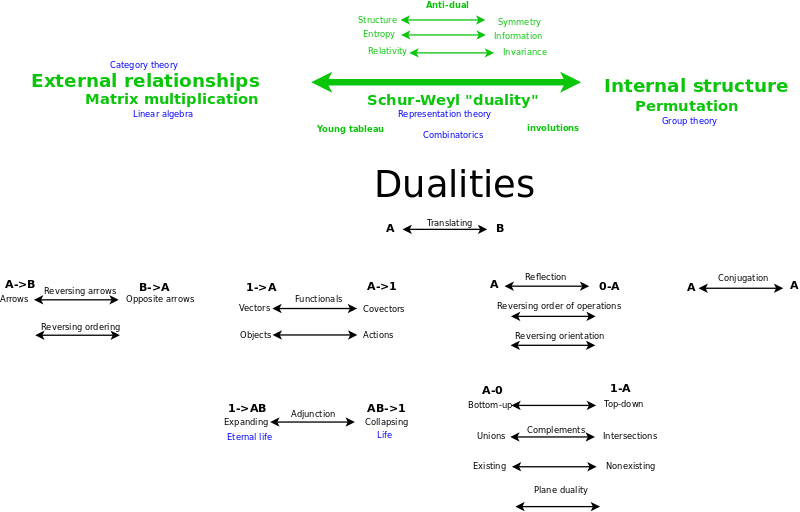The cognitive bottleneck which I describe above seems to be near the very heart of the connection betwen Lie groups and Lie algebras. Now I am trying to understand the implications by working them out for both the Lie groups and for the Lie algebras. I keep in mind that it may turn out that the Lie machinery is an accident of history and not the most profound way of describing what is going on at the deepest level. For example, it may or may not turn out that cognitively the exceptional Lie groups are spurious. I will list some connections that I am trying to make and understand or conclude otherwise.

I need to understand the link between the simple roots and the conjugate transposes (real, complex, quaternion) and the inner products (real, complex, quaternion) which the Lie groups preserve and are thus defined by. Apparently, the most ordinary Lie group {$A_{n}$} is defined by the complex inner product (rather than, say, the real inner product). This means that the complex inner product based on two dimensions is the most ordinary. I imagine it may be expressing the duality of the options of counting forwards and backwards. The symplectic group is defined by the quaternion inner product and this would make sense if we notice that it is establishing two sequences, thus making for four dimensions. The orthogonal groups are defined by the real inner product and they can be thought of as carrying a single dimension because they are counting only one side of the sequence, outside (when there is an external mirror?) or inside (when there is an internal mirror?). Taken together the options define 2 + 4 + 1 + 1 = 8 dimensions. And this brings to mind the Bott periodicity clock or the Clifford algebra clock. I also have an 8-cycle in my study of cognitive frameworks.

I would like to try to relate the Lie groups with four geometries: affine, projective, conformal and symplectic. And perhaps more basically, with four ways of thinking about a triangle:

• The concatenation of three paths.
• The intersections of three lines (on which we travel backwards and forwards).
• Three angles that divide the triangle.
• The oriented area of the triangle that we sweep out as we go around it.

I want to imagine that these get expressed as affine, projective, conformal and symplectic geometries, respectively. Certainly, I in my study of emotion and moods, I have found the different ways of thinking about a triangle to useful in grounding six transformations which take us from a less specified geometry to a more specified one. I need to learn what gets preserved and how ({$A_{n}$} - volume, {$C_{n}$} - oriented surface area?, {$B_{n}$} and {$D_{n}$} - distance. I am thinking that complex derivatives (that is, holomorphic functions where the first derivative is nonzero) preserve angles and so {$A_{n}$} expresses conformal geometry. And I am imagining that of {$B_{n}$} and {$D_{n}$}, perhaps the first has an explicit origin and is thus the basis for projective geometry, whereas the second does not have an explicit origin and is the basis for affine geometry.

Finally, I am trying to think of the root systems as constructing infinite families of polytopes. Here it may be helpful to identify each eigenvalue with a vertex and each root with an edge of a polytope. We have the following kinds of building blocks: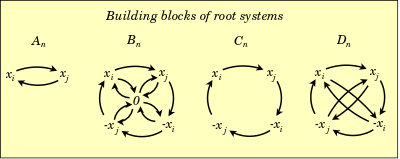{$A_{n}$} can be identified with the simplexes. I think of the simplex as having a unique center - the -1 simplex - which keeps moving as the simplex grows. Similarly, the simplex has a unique volume. The binomial expansion then codes for all of the subsimplexes.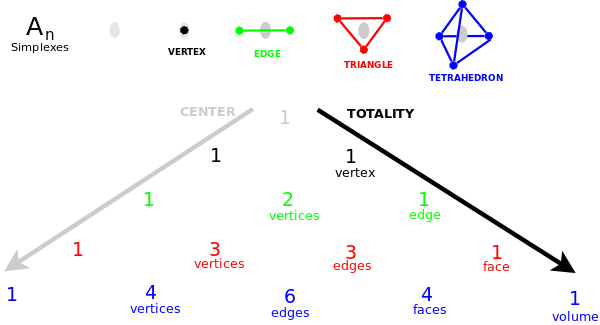Now I think of the cross-polytopes are generated by the simplex creating pairs of vertices, positive and negative, which are linked to all of the existing vertices but not to each other. This accords with the root system {$C_{n}$}. If we look at the triangle which counts the sub-polytopes, then we see that it has a unique center but the largest number counts the surface area, which is to say, there is no volume!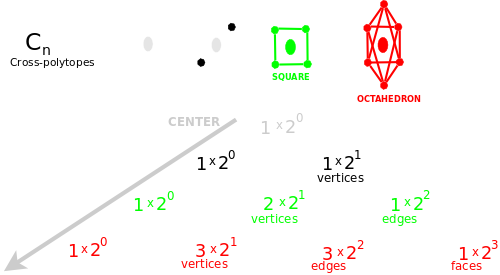The duals of the cross-polytopes are the hypercubes, which can be thought of as generated by mirrors (hyperplanes). They have a volume but no center! I don't see how to relate {$B_{n}$} with such a hypercube. Although perhaps the 0 is the total volume and it lets us construct our hypercube "top down" by dividing the whole volume rather than "bottom up" by assembling the vertices.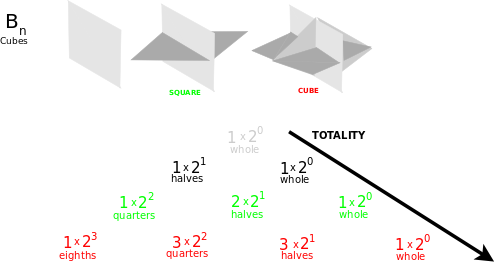Finally, I expect there should be an infinite family of polytopes {$D_{n}$} with no center and no volume.

Parsiųstas iš http://www.ms.lt/sodas/Book/20180207Attempt
Puslapis paskutinį kartą pakeistas 2018 gruodžio 09 d., 20:33